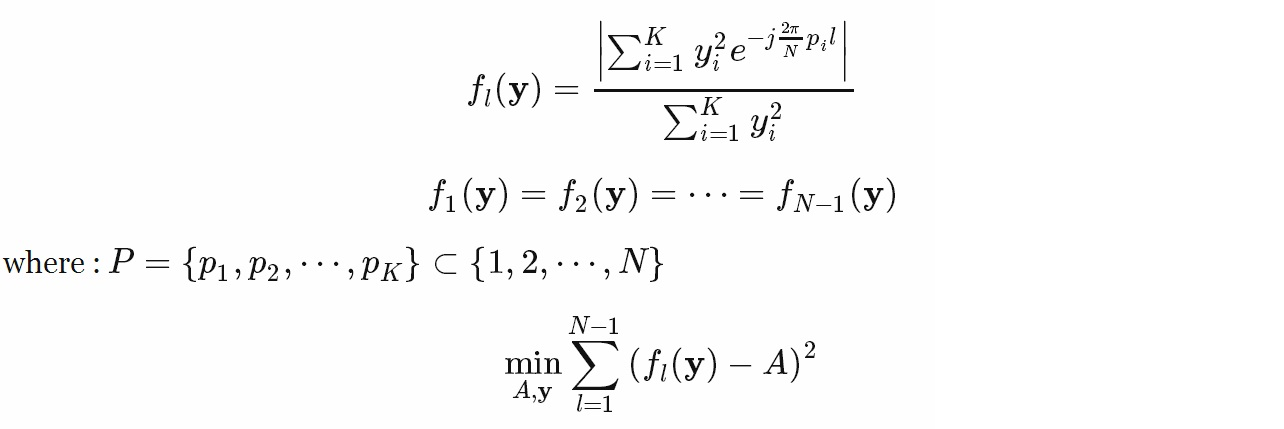# What numerical optimization method is useful?

 0 I'm facing this overdetermined system of equations and I'm looking for a numerical optimization method to solve it by minimizing and using least square method. I don't know how to write some formula here so I wrote it in mathjax and copied the picture here:A is the value of f_1(y) = f_2(y) = ... = f_N-1(y) =A. Is this minimization suitable for solving the system of equations? Do you have any suggestions? Which numerical method is suitable for solving this minimization if it is useful? Thanks asked 28 Jul '13, 12:49Mahdi Khosravi 1 accept rate: 0%fbahr ♦ 4.6k●7●16 I assume $$j=\sqrt{-1}$$ and $$\left|\cdot\right|$$ is complex modulus? That being the case, is $$y\in\mathbb{R}^K$$ or is $$y\in\mathbb{C}^K$$? (28 Jul '13, 17:11) Paul Rubin ♦♦
Be the first one to answer this question!
 toggle preview community wiki

By Email:

Markdown Basics

• *italic* or _italic_
• **bold** or __bold__
• image?![alt text](/path/img.jpg "Title")
• numbered list: 1. Foo 2. Bar
• to add a line break simply add two spaces to where you would like the new line to be.
• basic HTML tags are also supported

Tags: